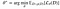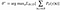# An Introduction to Meta-Learning

At Walmart Labs, we utilize meta-learning every day — whether it’s in our robust item catalog or item recommendations. This article will walk through what meta-learning is and how it is being used to solve practical industry problems.

Meta-learning is an exciting area of research that tackles the problem of learning to learn. The goal is to design models that can learn new skills/examples or quickly modify to new environments with minimal training examples. Not only does this dramatically speed up and improve the design of Machine learning (ML) pipelines or neural architectures, but it also allows us to replace hand-engineered algorithms with novel approaches learned in a data-driven way (Vanschoren, 2018).

The goal of meta-learning is to train a model on a diverse set of tasks, such that it can solve new tasks with only a handful of training samples. It tends to focus on finding model agnostic solutions, whereas multi-task learning remains deeply tied to model architecture.

Thus, meta-level AI algorithms make AI systems:

· Learn faster

· Adaptable to environmental changes like in Reinforcement Learning

One can solve any type of problem with a single model, but meta-learning should not be confused with one-shot learning.

# Problem Overview

Each task is associated with a dataset D, containing both feature vectors and true labels. A good meta-learning model should be trained for a diverse set of tasks and optimized for the best performance based on the probability distribution of different tasks, p(D), where p(D) includes new/unseen tasks too. The optimal model parameters are:Figure 1: Find parameter 𝜽* which minimizes the expected loss where 𝜽* is the optimal weight to infer. Here, one dataset is considered as one training sample.

Let’s split dataset D into two parts, a support set S (for the meta-learner) for learning, and a prediction set B (the bottom-level models) for training and validation, D = ⟨S,B⟩. Dataset D contains pairs of feature vectors and labels, D = {(xi,yi)} and each label belongs to a known label set, L. Let’s say, our classifier fθ with parameter θ outputs a log probability of a data point belonging to the class y, given the feature vector x, Pθ(y|x).

The optimal parameters maximize the probability of true labels across multiple batches B⊂D:Figure 2: Find the parameter 𝜽* which maximizes the expected sum of log probability.

We would like to make the training process replicate what happens during inference. Hence, we would like to “imitate” datasets with a subset of labels to avoid revealing all the labels to the model. Thus, changing the optimization procedure to encourage fast learning:

1. Sample a subset of labels, L

2. Sample a support set, SL ⊂ D and a training batch, BL ⊂ D. These sets contain data with labels belonging to the sampled label set L

3. The support set is part of the input model

4. The final optimization uses mini-batch BL for computing losses and updating model parameters via backpropagation.

So, we treat (SL, BL) as one data point. Symbols in red are added for meta-learning in addition to the supervised learning objective:

The idea is, similar to using a pretrained model in image classification (ImageNet) or language modeling (big text corpora) when only a limited set of task-specific data samples are available. Meta-learning, rather than fine-tuning according to one down-stream task, optimizes the model to perform well at countless tasks (Weng, 2018).

# Practical, Relevant Industry Problems

## Use Case 1: Placeholder detection in images

Common problems with products missing an image.

We may have multiple models for doing n number of tasks based on classification, regression, and Reinforcement Learning tasks based on Q-learning, Double Q-learning, etc. Now, given an image to check if it is a placeholder or not, the meta-learner being the top-level AI model will try to infer the predicted value from the bottom-level AI models, like models built on image classification, image-based QA to Reinforcement Learning tasks based on Q-learning, Double Q-learning, etc. Let’s say our meta-learner gives a probability number of whether the image is a placeholder or not.

Now, the meta-learner acts like a student and rewards itself if the predicted result is correct. If it’s not correct, it is penalized by the teacher (the actual value). Hence, with the help of just one example, your meta-learner tries to learn and gradually provides the correct results. This is an example of few-shot learning that does not require labeled data.

## Use Case 2: Fraudulent transaction detection

We can use meta-learner to detect fraudulent transactions based on other tasks which the low-level models were trained on.

Meta-learning could be used to resolve the use cases we mentioned above when there are only 10 to 100 training examples. The top-level model tunes the bottom-level models (each model could be made from a different task) to extract knowledge from them. Then, it predicts the results used to create new training examples. If its prediction is correct, then the teacher would reward it; otherwise, it is penalized. In this situation, the teacher refers to the optimizer to penalize the weights of the top-level model (student).

So, meta-learning takes knowledge from previous tasks to create a solution for the current task. Thus, it aids in optimizing the low-level AI model’s architecture, hyperparameters, and dataset tuning.

Try out demo code for meta-learning.

# Mathematical Background for Meta-Learning

Another popular view of meta-learning separates the model update into two stages:

· A classifier, fθ, is the “student” model trained to complete a given task

· In the meantime, an optimizer, gϕ(teacher model), learns how to update the “student” model’s parameters via the support set S, θ′ = gϕ(θ,S)

Thus, in the final optimization step, we need to update both θ and ϕ to maximize:

In the figure below, we show a simple model and a task-agnostic algorithm, Model-Agnostic Meta-Learning (MAML) that trains a model’s parameters such that a small number of gradient updates will lead to fast learning on a new task. It can be readily applied to regression, classification, and even Reinforcement Learning (RL).

Suppose we are seeking to find a set of parameters θ that are highly adaptable. During the course of meta-learning (the bold line), MAML (Model Agnostic Meta-Learning) optimizes for a set of parameters such that when a gradient step is taken with respect to a particular task i (the gray lines), the parameters are close to the optimal parameters θ*(i) for task i.

## How to Learn These Meta-Parameters

We now have two nested training processes: the meta-training process of the optimizer/meta-learner in that the (meta-)forward pass includes several training steps of the model (with forward, backward, and optimization steps).

Here we won’t have an error signal that has to be reduced between the predicted and the actual label.

We would like a meta-loss that is indicative of how well the meta-learner is performing its task, training the model.

So, in order to calculate meta-loss, we will need a hand-defined optimizer like Stochastic Gradient Descent (SGD) or Adaptive Moment Estimation(ADAM). Meta-loss can be defined as the sum of all losses computed during the training of various lower-level models.

Meta-loss is basically comprised of the following updates:

1. Second-Order Derivatives: Backpropagating the meta-loss through the model’s gradients involves computing derivatives of a derivative (i.e., second derivatives). But, in practice, we often drop the second derivatives and only backpropagate through the model weights to reduce the complexity.

2. Coordinated Sharing: Here, we design the optimizer for a single parameter of the model and duplicate it for all of the parameters (i.e., share its weights along the input dimension associated with the model parameters). This way the number of parameters of the meta-learner is not a function of the number of parameters of the model.

When the meta-learner is a network with a memory like a Recurrent Neural Network (RNN), we can still allow having a separate hidden state for each model parameter to keep separate memories of the evolution of each model parameter.

In short, meta-learning produces a versatile AI model that can learn to perform various tasks without having to train them from scratch. Meta-learning is not limited only to semi-supervised tasks, it can be extended to tasks like item recommendation, importance sampling (density estimation), and reinforcement learning tasks. Every time we try to learn a task, we gain experience regardless of if we were successful or not. Therefore, meta-learning allows us to refer to our past experiences to easily overcome new, different tasks without requiring a large knowledge base.

# References

2. Gregory Koch, Richard Zemel, and Ruslan Salakhutdinov Siamese neural networks for one-shot image recognition in ICML Deep Learning Workshop. 2015.

3. Adam Santoro, et al. Meta-learning with memory-augmented neural networks in ICML. 2016.

4. M. Andrychowicz et. al in Learning to learn by gradient descent by gradient descent in NIPS 2016.

5. Thomas Wolf, Julien Chaumond & Clement Delangue in META-LEARNING A DYNAMICAL LANGUAGE MODEL in ICLR 2018 workshop.

6. Chelsea Finn, Pieter Abbeel & Sergey Levine in Model-Agnostic Meta-Learning for Fast Adaptation of Deep Networks in EMNLP 2018.

Written by

Written by

## Ashish Gupta

#### Catalog Data Science Team @WalmartLabs